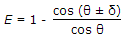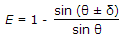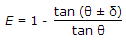# Civil Engineering - Surveying

### Exercise :: Surveying - Section 1

21.

In chain surveying field work is limited to

 A. linear measurements only B. angular measurements only C. both linear and angular measurements D. all the above.

Explanation:

No answer description available for this question. Let us discuss.

22.

Two concave lenses of 60 cm focal length are cemented on either side of a convex lens of 15 cm focal length. The focal length of the combination is

 A. 10 cm B. 20 cm C. 30 cm D. 40 cm

Explanation:

No answer description available for this question. Let us discuss.

23.

If θ is the vertical angle of an inclined sight, δ is the angle of tilt of the staff, the error

 A.B.C.D. none of these.

Explanation:

No answer description available for this question. Let us discuss.

24.

One of the tacheometric constants is additive, the other constant, is

 A. subtractive constant B. multiplying constant C. dividing constant D. indicative constant.

Explanation:

No answer description available for this question. Let us discuss.

25.

The limiting length of an offset does not depend upon

 A. accuracy of the work B. method of setting out perpendiculars C. scale of plotting D. indefinite features to be surveyed.